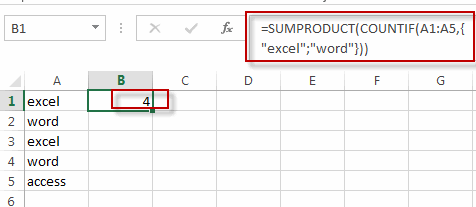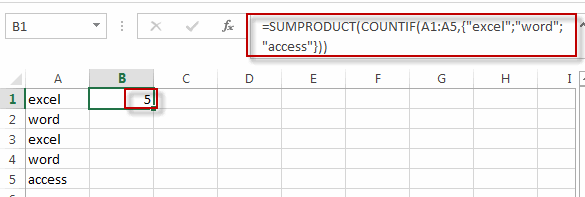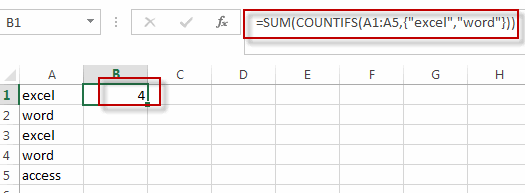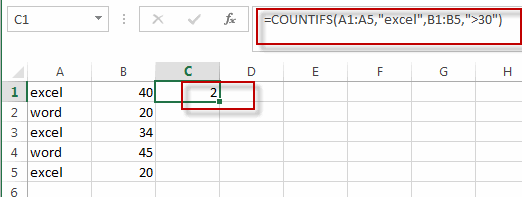# COUNTIF with Multiple Criteria

This post will guide you how to countif multiple criteria on the same column in excel. How do I countif multiple criteria on the different columns in excel. How to use COUNTIF to count cells that match multiple criteria on the same column or the different columns in Excel.

## COUNTIF with Multiple Criteria in the Same column

Assuming that you have a list of data in column A and you want to count the number of two specified text string (excel and word) in range A1:A5, how to achieve it.

To count the number based on multiple criteria in the same range or column in Excel, you can create a formula based on the SUMPRODUCT function and the COUNTIF function. Just like this:

`=SUMPRODUCT(COUNTIF(A1:A5,{"excel";"word"}))`

Then type this formula into a blank cell and then press Enter key in your keyboard to apply this formula.You will see that the count number is displayed in that cell.

And if you want to add one more criteria in this formula, for example, you need to count the number of three text strings in the given range, you just add that text string into the formula as below:

`=SUMPRODUCT(COUNTIF(A1:A5,{"excel";"word";"access"}))`Or you can also use another formula based on the SUM function and the COUNTIFS function to achieve the same result. Like this:

`=SUM(COUNTIFS(A1:A5,{"excel","word"}))`

Type this formula into a blank cell, and then press Enter key.## COUNTIF with Multiple Criteria in the Same column

Assuming that you have two lists of data in Range A1:B5, and one is the product name and another is sale values. And you want to count the number of product “excel” and its sale value is greater 30. How to achieve it. You can use the COUNTIFS function to create a formula to achieve the result.

Like this:

`=COUNTIFS(A1:A5,"excel",B1:B5,">30")`

Type this formula into a blank cell and then press Enter key.### Related Functions

• Excel COUNTIF function
The Excel COUNTIF function will count the number of cells in a range that meet a given criteria. This function can be used to count the different kinds of cells with number, date, text values, blank, non-blanks, or containing specific characters.etc.= COUNTIF (range, criteria)…
• Excel SUMPRODUCT function
The Excel SUMPRODUCT function multiplies corresponding components in the given one or more arrays or ranges, and returns the sum of those products.The syntax of the SUMPRODUCT function is as below:= SUMPRODUCT (array1,[array2],…)…
• Excel SUM function
The Excel SUM function will adds all numbers in a range of cells and returns the sum of these values. You can add individual values, cell references or ranges in excel.The syntax of the SUM function is as below:= SUM(number1,[number2],…)…
• Excel COUNTIFS function
The Excel COUNTIFS function returns the count of cells in a range that meet one or more criteria. The syntax of the COUNTIFS function is as below:= COUNTIFS(criteria_range1, criteria1, [criteria_range2, criteria2]…)…